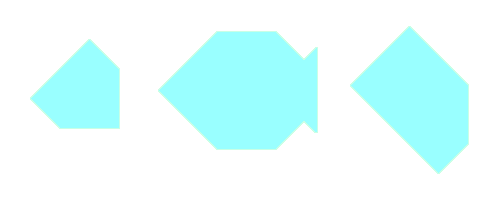# Tangrams

Objective: Classify angles in a variety of orientations according to whether they are right angle, less than right angle, or greater than right angle. Identify and sort specific quadrilaterals, including squares, rectangles, parallelograms and trapezoids.
Materials: Tangrams.Answer the following questions: How many tangram pieces have at least one right angle? How many tangram pieces have angles which are larger than right angles? How many tangram pieces have more than one right angle? How many tangram pieces have one or more angles smaller than a right angle? Build a square using any 2 pieces. Now try to build one using 3, then 4, 5, 6 and 7 pieces. Build a rectangle (squares don't count here!) using any 2 pieces. Now try using any 3, then 4, then 5, 6 and 7 pieces. How many different sized trapezoids can you build?

Objective: Cover a given 2-D shape with tangram pieces.
Materials: Tangrams, silhouette page.

 Create each of the following shapes using any or all of the seven tangram pieces.Create a silhouette puzzle for a friend to solve.Objective: Sort quadrilaterals and regular polygons according to the number of lines of symmetry.
Materials: Tangrams.Build each of the following quadrilaterals using your tangram pieces: sqauare, rectangle, kite, parallelogram, rhombus, trapezoid, quadrilateral. Determine the number of lines of symmetry for each of these figures. Try to build each in more than one way or in more than one size if possible. Determine the number of lines of symmetry in each shape. What do you notice? Build a 4-sided figure with no lines of symmetry. How do you know it has no lines of symmetry?